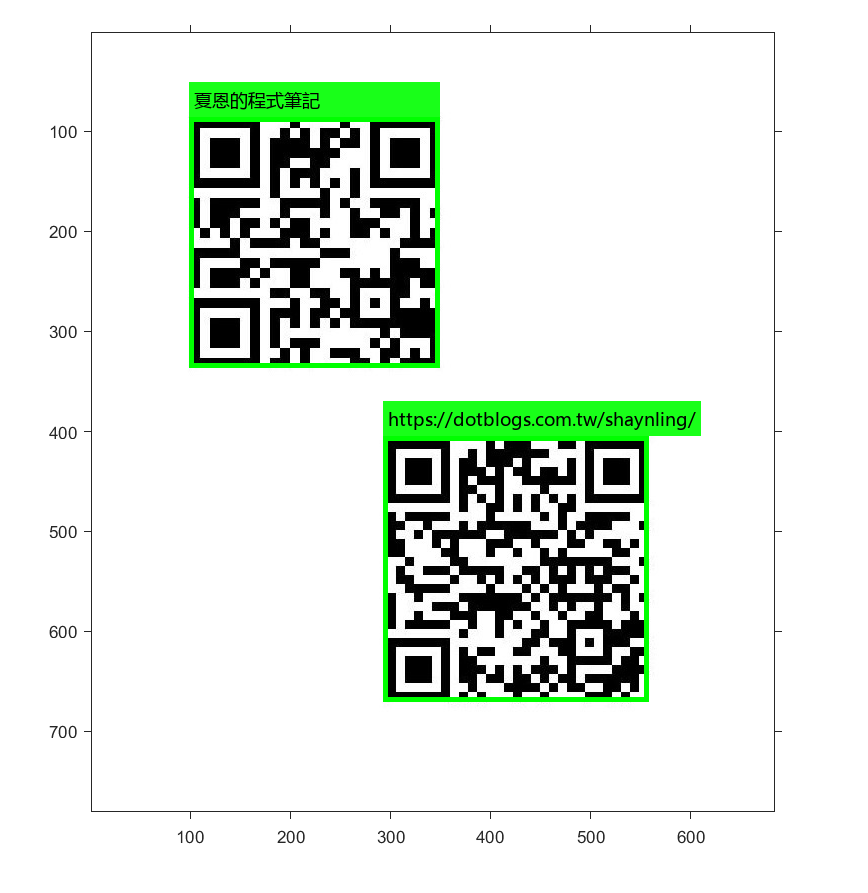### 【Mixing】在 MATLAB 中調用 Python 解析 QR code

• 1053
• 0
• 2019-01-20

MATLAB      Version 9.5      (R2018b)
Python         Version 3.5      (Based on anaconda)
- pyzbar     Version 0.1.7   (Install from pip)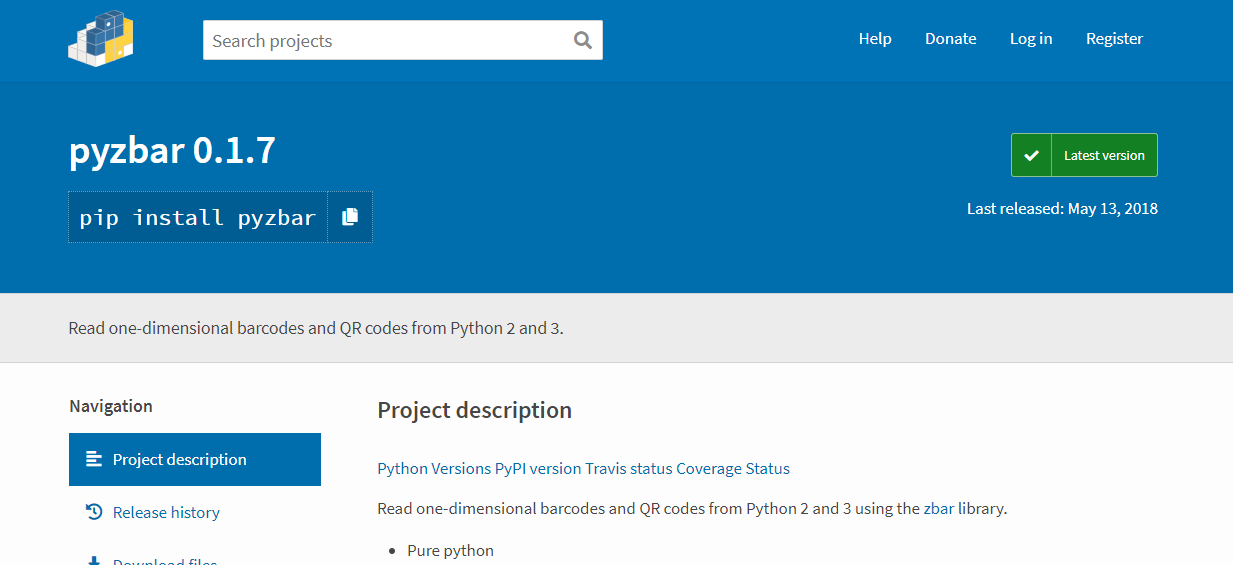## 用 Python 解析 QR code

# -*- coding: utf-8 -*-
"""
Created on Sat Jan 19 2019
@author: Shayne
"""

import numpy as np
import pyzbar.pyzbar as zbar

def detect(I):
# 偵測所有的 QR code
barcodes = zbar.decode(I)

# 逐一解碼，回傳位置與結果
bbox = []; msg = [];
for i, barcode in enumerate(barcodes):
bbox.append(np.array(barcode.rect))
msg.append(barcode.data.decode('utf-8'))

return bbox, msg

# -*- coding: utf-8 -*-
"""
Created on Sat Jan 19 2019
@author: Shayne
"""

import cv2
import numpy as np
import pyzbar.pyzbar as zbar
import matplotlib.pyplot as plt

def detect(I):
# 偵測所有的 QR code
barcodes = zbar.decode(I)

# 逐一解碼，回傳位置與結果
bbox = []; msg = [];
for i, barcode in enumerate(barcodes):
bbox.append(np.array(barcode.rect))
msg.append(barcode.data.decode('utf-8'))

return bbox, msg

plt.imshow(I)
plt.show()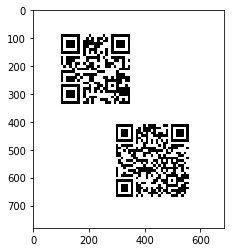bbox, msg = detect(I)
for rect in bbox:
c, r, w, h = rect
cv2.rectangle(I, (c, r), (c+w, r+h), (0, 255, 0), 5)

print(msg)

plt.imshow(I)
plt.show()

['https://dotblogs.com.tw/shaynling/', '夏恩的程式筆記']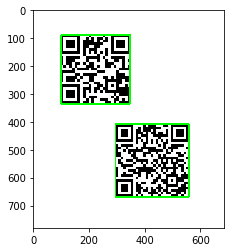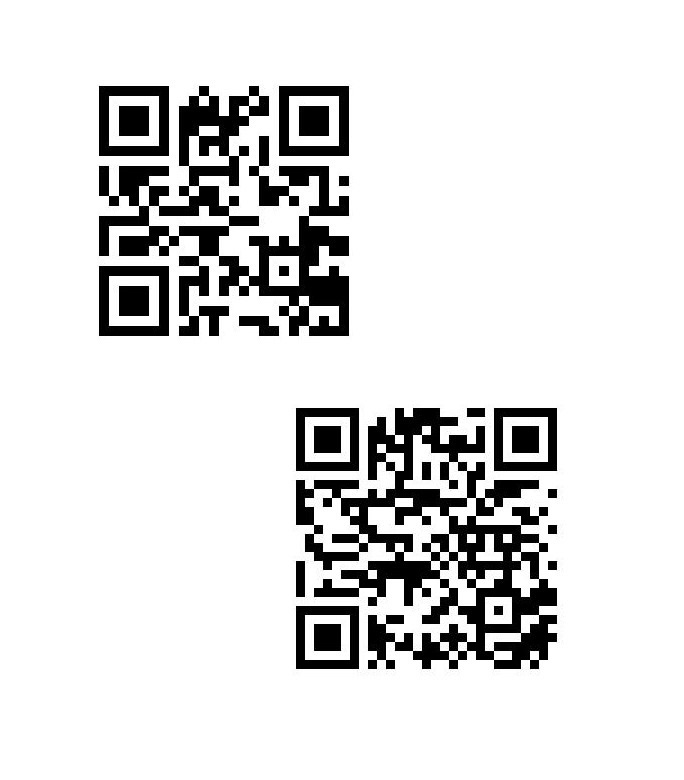## 設定 MATLAB 工作環境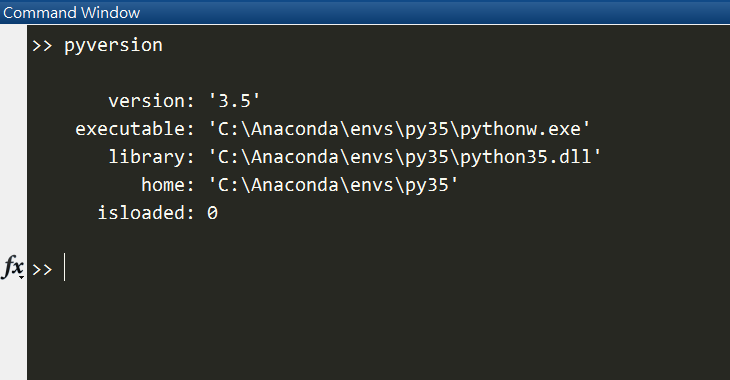(請注意：若要再次切換須重新啟動 MATLAB。)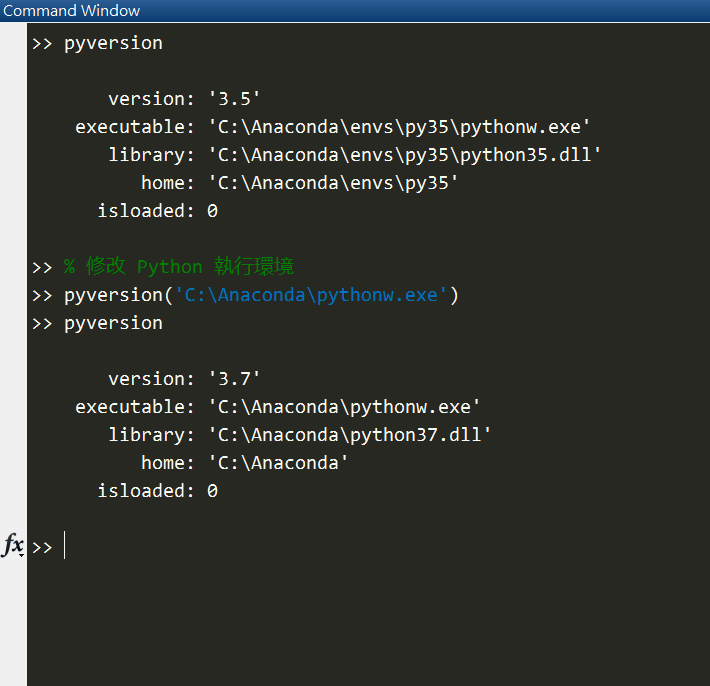## 牛刀小試

### 1. 資料型態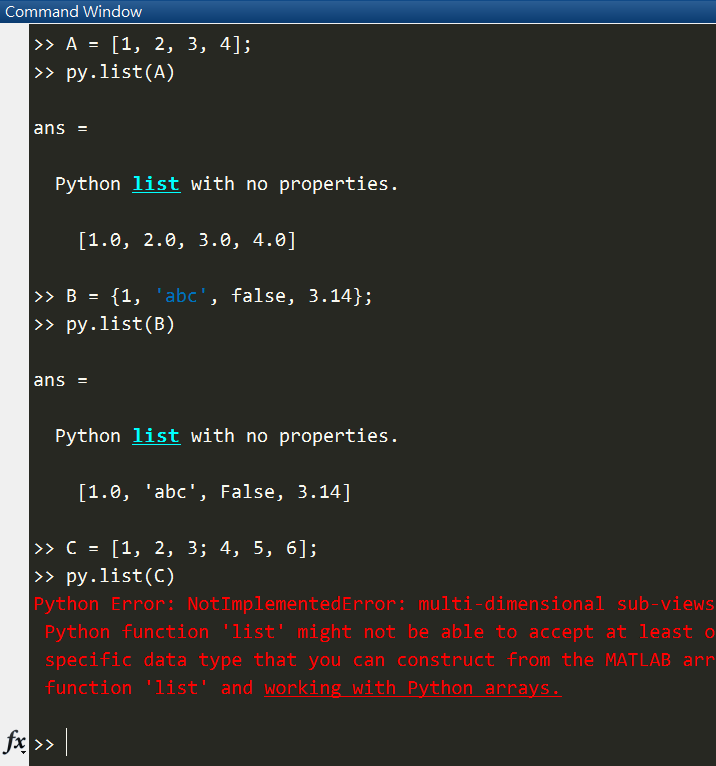### 2. 陣列運算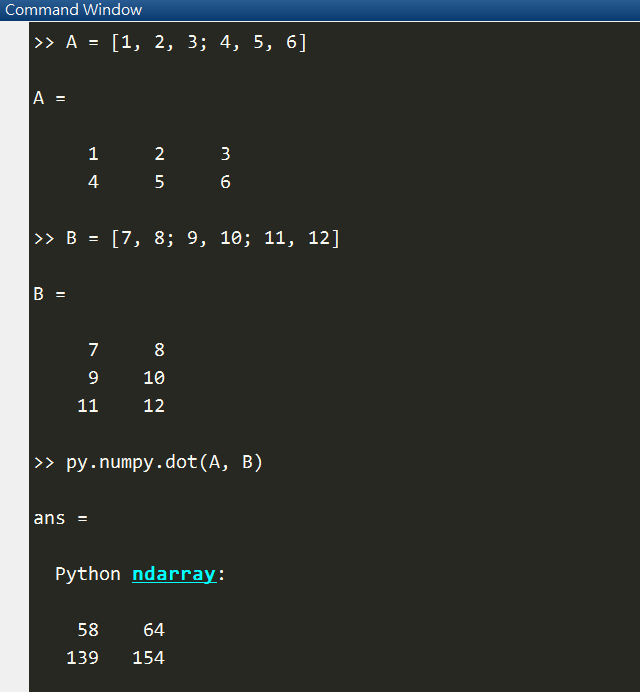### 3. 讀取檔案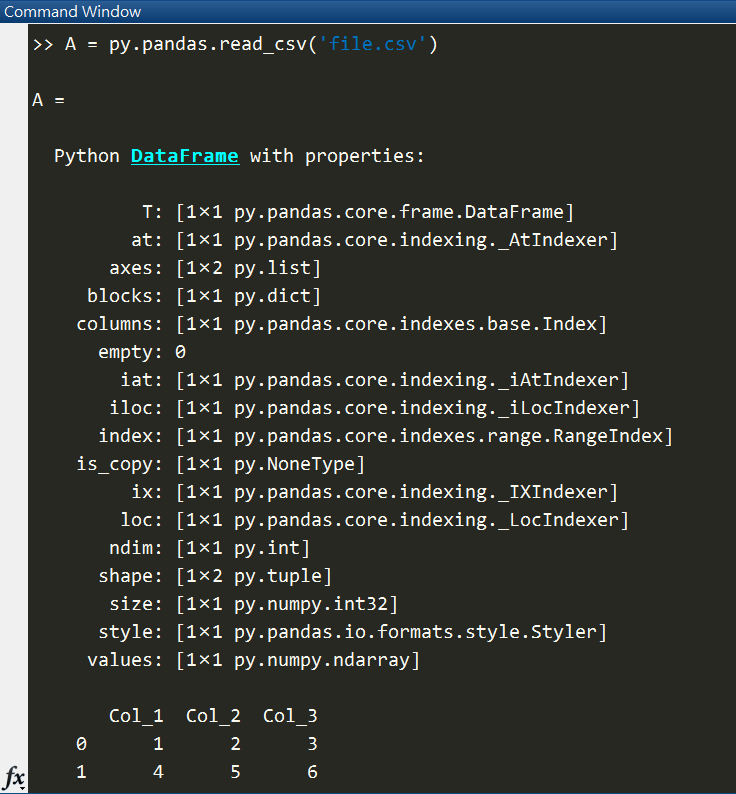## 主程式登場

MATLAB 的陣列是 column-major；而 Python 預設則是 row-major。

(夏恩幹過這種蠢事，花了好幾個小時呢...)

% MATLAB 調用 Python 範例程式
%
% Shayne, 2019.01.19

% 讀檔

% 轉換成 numpy.array 的型態
I1 = py.numpy.array(I);

% 呼叫 Python 函數
result = py.myfcn.detect(I1);
disp(result)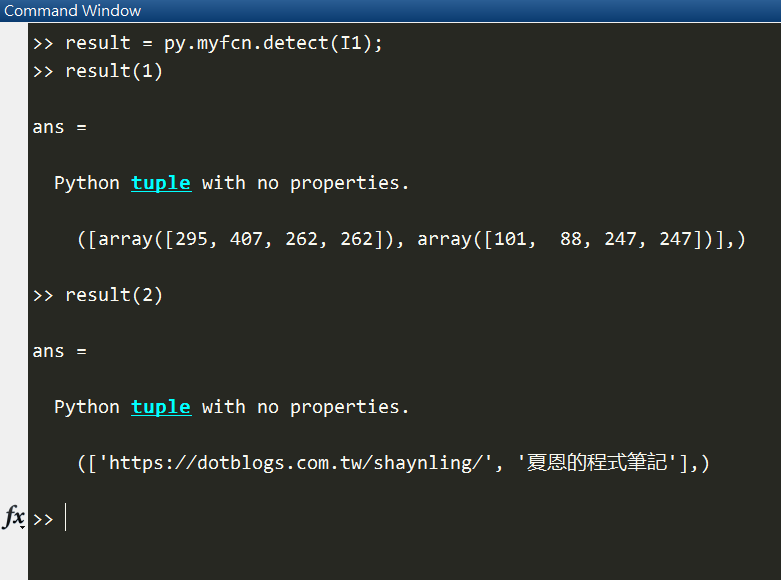1. 不論回傳值有幾個，在 MATLAB 中只能寫一個。
2. 不論回傳值是什麼型態，統一規定為 tuple

% 解析結果
R = cell(result);

% 計算回傳值中包含多少資料，不能直接寫 length(R)
N = length(R{1});

bbox = zeros(N, 4);
msg  = cell(N, 1);

for i = 1:N

% 使用 double() 轉換 array List 為 double
bbox(i, :) = double( R{1}{i} );

% 使用 char() 轉換 string List 為 char array
msg{i} = char( R{2}{i} );

end

% 出圖
I2 = insertObjectAnnotation(I, 'rectangle', bbox, msg, ...
'Color', 'g', ...
'LineWidth', 5, ...
'TextBoxOpacity', 0.9, ...
'FontSize', 18 );
imshow(I2)